Name:    Chapter 1 Test

Multiple Choice
Identify the choice that best completes the statement or answers the question.

Find the absolute value of the integer.

1.

–9
 a. | –9 | c. 9 b. –9 d. | 9 |

2.

14
 a. | –14 | c. 14 b. –14 d. | 14 |

3.

–190
 a. –190 c. 190 b. | 190 | d. | –190 |

4.

4 + (–3)
 a. 7 c. 1 b. –1 d. –43

5.

0 + (–17)
 a. –170 c. 17 b. –17 d. 0

6.

The temperature is –5°F at 6 A.M. During the next four hours, the temperature increases 17°F. What is the temperature at 10 A.M.?
 a. –12°F c. –22°F b. 22°F d. 12°F

Subtract.

7.

5 – 9
 a. 4 c. –4 b. –14 d. –59

8.

3 – (–7)
 a. 10 c. –10 b. –4 d. 4

9.

–11 – 15
 a. 4 c. 26 b. –4 d. –26

Multiply.

10.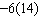a. –84 c. –70 b. –74 d. 84

11.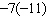a. 77 c. –77 b. 66 d. 88

12.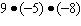a. 364 c. 362 b. 360 d. –360

Evaluate the expression.

13.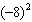a. 64 c. 16 b. –16 d. –64

Evaluate the expression.

14.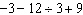a. –4 c. 4 b. –2 d. 2

15.

How many quadrants are in a coordinate plane?
 a. 2 c. 1 b. 4 d. 3

Write an ordered pair corresponding to the point.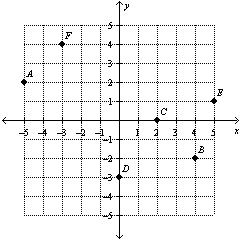16.

Point C
 a. (0, –2) c. (0, 2) b. (2, 0) d. (–2, 0)

Numeric Response

1.

Your bank account has a balance of –\$19. You deposit \$35. What is your new balance?

Use mental math to solve the equation.

2.

6 – b = –5

Tell how the Commutative and Associative Properties of Addition can help you find the sum mentally. Then find the sum.

1.

8 + (–13) + (–8)

2.

A quotient is undefined. What does this mean?